# Change tab color based on a cell value in Excel

Wouldn’t it be great to change the color of a worksheet tab based on a calculation?  If you’re not convinced, here are some possible scenarios:

• When a schedule reconciles or includes a specific cell value
• If there is an error in a range of cells
• To color code tabs to match a contents page

You were unsure at first, weren’t you?  But now you think it would be a good idea, don’t you?  The good news is, it’s pretty simple.

It just requires a small amount of code in a User Defined Function.

I didn’t think up this idea by myself.  The idea came from David Hager’s post, check out his post here.

## Creating the User Defined Function

To create a User Defined Function click Developer -> Visual Basic (or shortcut ALT + F11).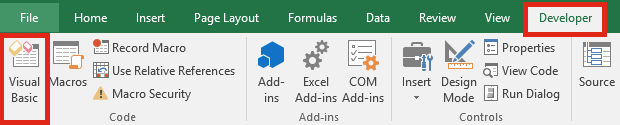The Visual Basic Editor window will open, click Insert -> ModuleAdd the code as shown below.  The code for User Defined Functions must be within a standard module to work correctly.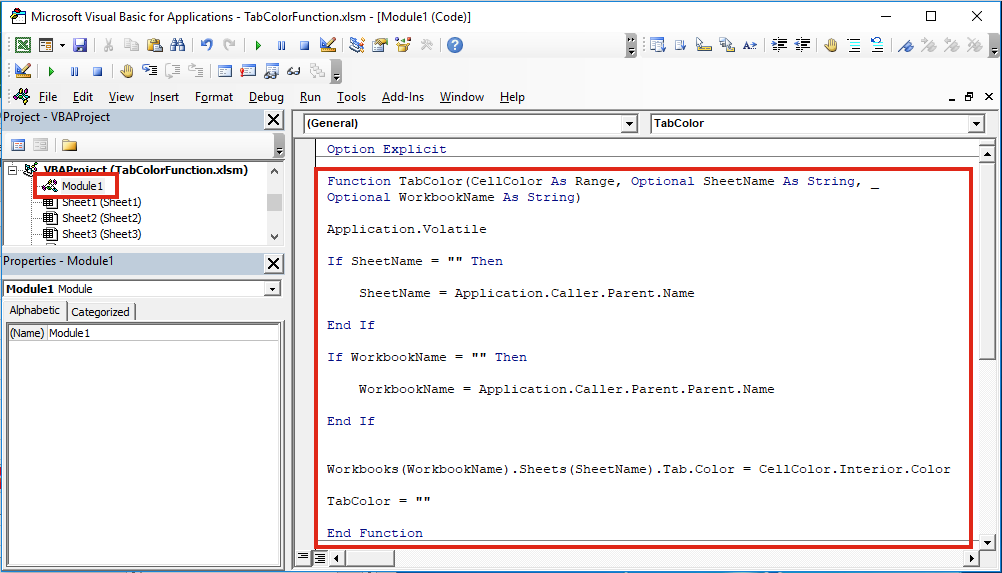VBA Code:

```Function TabColor(CellColor As Range, Optional SheetName As String, _
Optional WorkbookName As String)

Application.Volatile

If SheetName = "" Then
SheetName = Application.Caller.Parent.Name
End If

If WorkbookName = "" Then
WorkbookName = Application.Caller.Parent.Parent.Name
End If

If CellColor.Interior.Color = xlNone Then
'If cell color is no fill then make tab color automatic
Workbooks(WorkbookName).Sheets(SheetName).Tab.Color = xlAutomatic
Else
'Else change to the cell color
Workbooks(WorkbookName).Sheets(SheetName).Tab.Color = CellColor.Interior.Color
End If
TabColor = ""

End Function```

Depending on how you wish the User Defined Function to operate, there are a variety of ways to use this function.  See the examples in the section below.

## Using the Function

The Function we created above is now available within Excel for use, just like any other function.  The syntax is as follows:

`=TabColor(CellColor,[SheetName],[WorkbookName])`
• CellColor is a reference to a cell containing the color to be used
• SheetName is an optional argument to apply the function to another sheet in the same workbook
• WorkbookName is an optional argument to apply the function to another sheet in a different workbook

Now, let’s look at some examples.

### Example #1 – Basic usage

This examples shows the basic usage of the TabColor User Defined Function.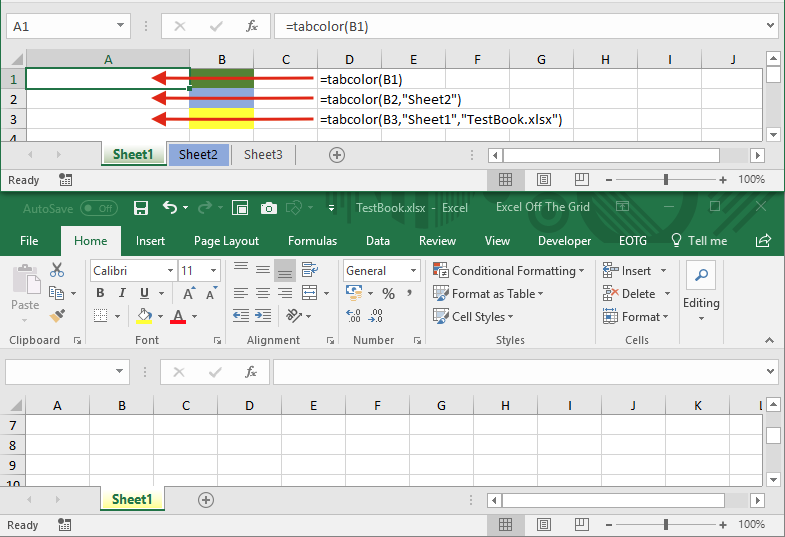Example 1a

Cell A1 contains the following formula:

`=TabColor(B1)`

The tab color in Cell B1 is applied to the sheet within which the function is used.

Example 1b

Cell A2 contains the following formula:

`=TabColor(B2,"Sheet2")`

The tab color in Cell B2 is applied to Sheet2 in the same workbook.

Example 1c

Cell A3 contains the following formula:

`=TabColor(B3,"Sheet1","TestBook.xlsx")`

The tab color in Cell B3 is applied to Sheet1 of the TestBook.xlsx workbook (which needs to be open for the function to work).

### Example #2: Where a cell includes a specific cell value

This example shows how to set the tab color based on a cell value.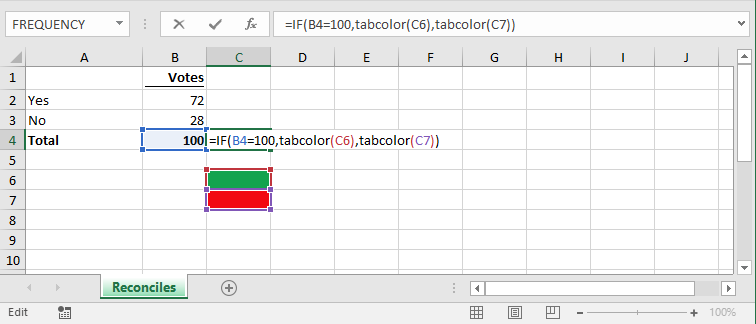The formula in cell C4 is:

`=IF(B4=100,tabcolor(C6),tabcolor(C7))`

Where the Total equals 100 the tab color is green, otherwise it is red.

### Example #3: Error in a range

The example shows the tab color changing where an error exists within a range.The formula in Cell D2 is:

`=IF(SUMPRODUCT(--ISERROR(B2:B9))>=1,TabColor(D3),TabColor(D4))`

The SUMPRODUCT element of the formula, counts the number of errors.  As there is one error in Cell B7 the IF function will show the TRUE result, which is causing the TabColor function to turn the tab color red.

### Example #4: Color code tabs to a contents page

This example shows the Sheet Name being constructed from two cells.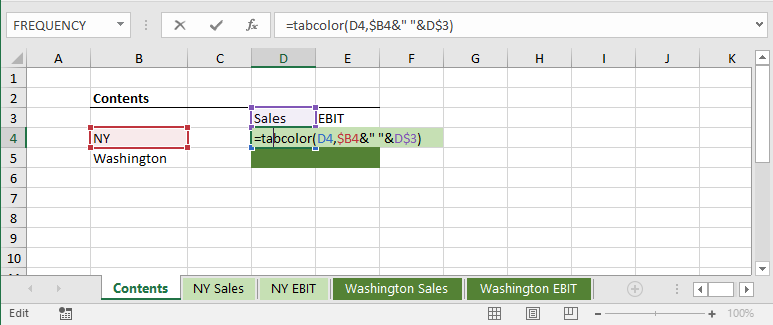The formula in Cell D4 is:

`=TabColor(D4,\$B4&" "&D\$3)`

NY in Cell B4 and Sales in Cell D3 are concatenated with the ” & ” symbol.  The TabColor function is causing the “NY Sales” tab to turn light green.

As the TabColor function is not using calculated values it is able to point to it’s own cell without causing a circular reference.

## Use RGB to apply the color

In the UDF above, the color is set by linking to a cell, by changing the code it is possible to use the RGB values instead.

```Function TabColorRGB(Red As Integer, Green As Integer, Blue As Integer, _
Optional SheetName As String, Optional WorkbookName As String)

Application.Volatile

If SheetName = "" Then
SheetName = Application.Caller.Parent.Name
End If

If WorkbookName = "" Then
WorkbookName = Application.Caller.Parent.Parent.Name
End If

Workbooks(WorkbookName).Sheets(SheetName).Tab.Color = RGB(Red,Green,Blue)

TabColor = ""

End Function```

This function can be used as follows:

`=TabColorRGB(255,0,0,"Sheet1","TestBook.xlsx")`

The first 3 arguments are the Red, Green and Blue color codes.  The Sheet name and Workbook name are both optional.  The formula above will turn the tab for Sheet1 of TestBook.xlsx to a red (which is the color of Red = 255, Green = 0 and Blue = 0)

## Conclusion

Changing the tab color using a formula is quite easy and can provide a simple visual representation of the status of a worksheet within the workbook.

Discover how you can automate your work with our Excel courses and tools.

The complete program for saving time by automating Excel.

Excel Automation Secrets
Discover the 7-step framework for automating Excel.

Office Scripts: Automate Excel Everywhere
Start using Office Scripts and Power Automate to automate Excel in new ways.

### 13 thoughts on “Change tab color based on a cell value in Excel”

1.This didn’t work for me

•I’ve just checked and all worked fine. Where did you place the code? It needs to be in a standard VBA module.

2.This works great in an IF formula but I’m wanting to use an IFS formula to change tab color depending on which of 3 or 4 options is selected. When I use the same idea as shown above but with the IFS formula, it changes the tab color to the last “TabColor” setting in the formula regardless of which argument is true. Any suggestions?

•Yes, you’re right… interesting. IF and IFS appear to function differently in regard to this. I wonder why?

3.Good morning,

Thanks for this function, it’s pretty cool.

I’m my case only works in the first case: “=TabColor(B1)”

If I include the sheet or insert the function in another function like “=IF(B4=100,tabcolor(C6),tabcolor(C7))” it didn’t works.

Do you know what can be happening?

Best regards,
Martín.

•Hi Martin – apologies, but I’ve not been able to re-create your issue:

I have tried the following and it still works for me:

`=IF(B3=1,TabColor(D3),TabColor(D4))`
•Hi,

I recheck everything and it was a spelling mistake. In my excel, the functions separator is the “;” instead of the “,” so my function has to be:

=IF(B3=1;TabColor(D3);TabColor(D4))

Thanks for the help!

4.You just helped me solve something I’ve been trying to figure out a code for off and on for almost 2 years! All I needed was a way to have the tab color update automatically depending on which quarter the sales took place in. I kept trying to make it work off different kinds of conditional formatting (no go because the relevant cell on the page is colored by nested conditionals based on IFS formula). With your system I was able to simply create a lookup to the Index page where the Quarter Sheet Headers were stored. This works beautifully when I copy the pages for each show. Thanks so so so so much!

•2 years!!! In which case, I’m glad I could help.

5.Is there a way to accomplish this without VBA? If a cell say A1 says “yes” the tab is red if A1 says “no” the tab is green and if A1 says nothing the tab color is nothing. Thanks

•The only way I know if is with VBA, unless you do it manually.

6.•